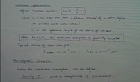### 주메뉴

### Computational Approach to Various Fluid Problems

• 한국과학기술원
• 이준상• 주제분류
공학 >토목ㆍ도시 >해양시스템학
• 등록일자
2009.07.17
• 조회수
6,626
•
Introduction to modern computational fluid dynamics. Finite difference and finite volume methods. Explicit, implicit, and iterative techniques. Solutions of elliptic, parabolic, and hyperbolic equations. Emphasis on algorithms and some engineering applications.
Introduction, Partial Differential Equations (1차시_01)#### 차시별 강의1.Introduction, Partial Differential Equations (1차시_01)Introduction, Partial Differential Equations (1차시_02)2.Basics of Finite Difference Methods, Stability (2차시_01)Basics of Finite Difference Methods, Stability (2차시_02)3.Wave Equation, Heat Equation (3차시_01)Wave Equation, Heat Equation (3차시_02)4.Laplace Equation, Burgers’ Equation (4차시_01)Laplace Equation, Burgers’ Equation (4차시_02)#### 연관 자료#### 사용자 의견

강의 평가를 위해서는 로그인 해주세요.

#### 이용방법

• 강의 이용시 필요한 프로그램 [바로가기]

※ 강의별로 교수님의 사정에 따라 전체 차시 중 일부 차시만 공개되는 경우가 있으니 양해 부탁드립니다.